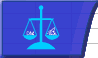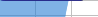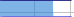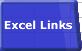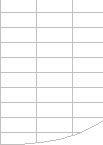# 'Calculate' always in the Status BarSmart Recalculation Evaluation Circumstances Calculation Process Dependency Trees Controlling Calculation "CALCULATE" in Status Bar Calculation Methods Calculating from VBA Volatile Functions & Actions User-Defined Functions Repetitive Calculation Version Timing Comparison## Dependency Tree Limits

There are two limits to the number of dependencies that Excel versions prior to Excel 2007 can track before it must do full calculations instead of recalculations.

• The number of different areas in a sheet that may have dependencies is limited to 65,536.
• The number of cells that may depend on a single area is limited to 8K.

After the workbook has passed these limits, Excel no longer attempts to recalculate only changed cells. Instead, it recalculates all cells at each calculation. These limits saves the substantial amount of memory required for the dependency trees. When this happens the status bar permanently shows CALCULATE?for all open workbooks even after closing the main workbook, until you close and reopen Excel.

If you find that recalculation is taking longer than full calculation, or the time taken to update the dependency tree at each change is too large, you can force each calculation to be a full one using Workbook.ForceFullCalculation from VBA.

I do not know of a way of counting the number of dependencies, so it is not easy to work out how to get below the limit, but:

• Large numbers of dependencies are usually caused by many formulae referencing large ranges using functions like OFFSET, INDEX, and the Lookup. You can reduce the number of dependencies by reducing the use of these functions, and also by reducing the size of the ranges they refer to. If you reduce the number of dependencies below the limits you have to save the workbook, close and restart Excel before Excel recognises that the number of dependencies has fallen below the limits.
• Excel 2002 allows you to do a full recalculation with dependency tree rebuild, but this does not alter the limits.
• When you have exceeded the dependency limitspressing F9 will cause a recalculation even when in Automatic mode.

Because there are other conditions that can cause "Calculate" to appear in the status bar, test for the other conditions::

• Turn off Iteration, press F9 and look for "Circular Reference" in the statusbar.
• Press Ctrl/Alt/F9 to do a full calculation. If you have hit the limit the status bar will show the calculation % climbing to and reaching 100%, and then being replaced by "Calculate"

For Microsoft's official view on the dependency limit see MSKB Article Q243495 "Calculate Message Remains in Status Bar If 65,536 Formula References"© 2001-2020 Decision Models Legal Disclaimer Privacy Statement